# NCERT Exemplar Class 9 Science Solutions for Chapter 3 - Atoms And Molecules

## NCERT Exemplar Solutions Class 9 Science Chapter 3 – Free PDF Download

NCERT Exemplar Class 9 Science Chapter 3 Atoms and Molecules are essential study materials for CBSE Class 9 students that play a pivotal role in providing a clear understanding of the concepts covered in Class 9 Atoms and Molecules. Students should follow the NCERT Exemplar Solutions provided here in order to score well in Class 9 examination and for a better understanding of chemistry-related topics in future.

Atoms and Molecules of NCERT Exemplar Class 9 Science is prepared as per the latest CBSE Syllabus 2021-22 and has answers to the questions provided in NCERT Exemplar book. This page has answers to 10 Multiple choice questions, 13 short answer questions and 25 long answer questions which will help you comprehend with the questions that can be asked in the examination.

### Download PDF of NCERT Exemplar for Class 9 Science Chapter 3 – Atoms and Molecules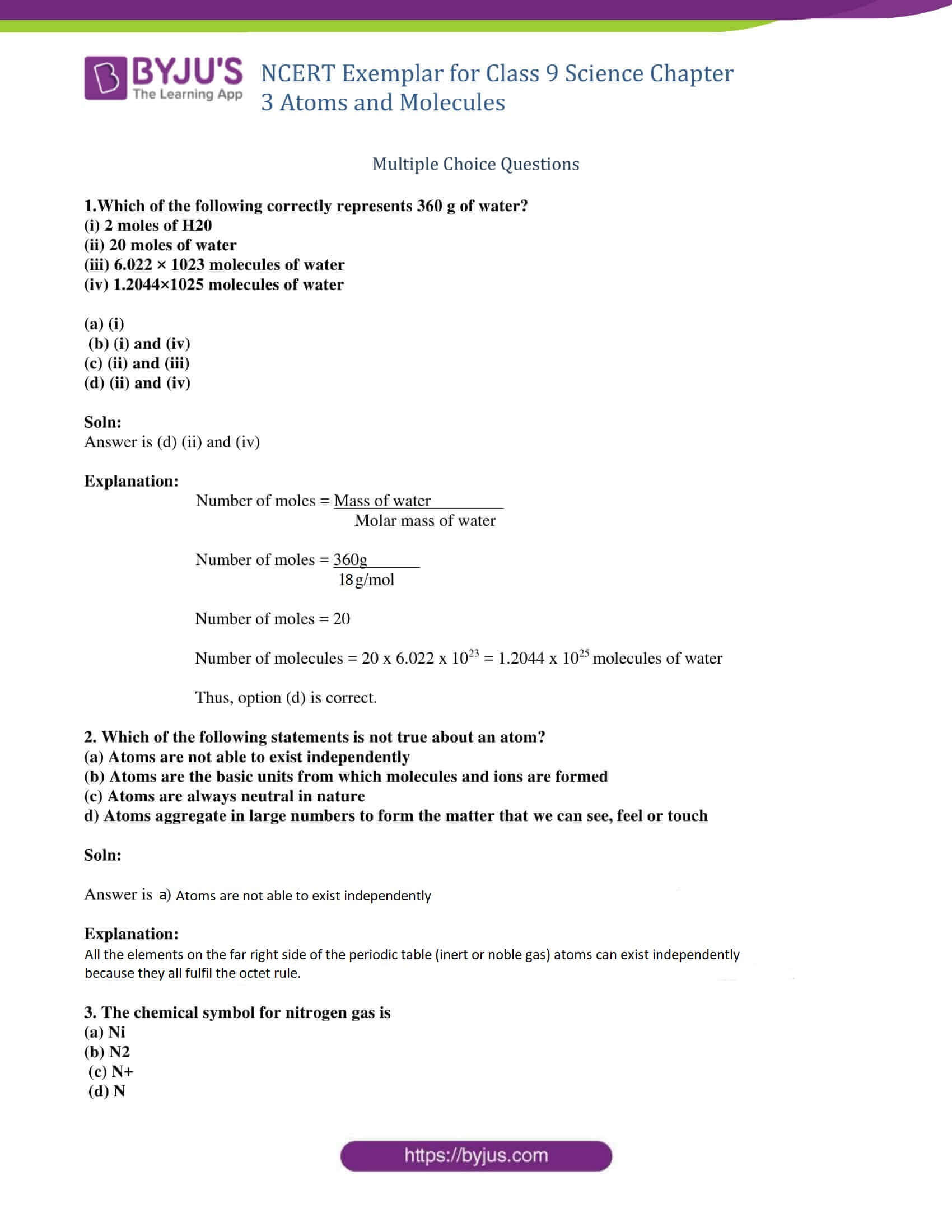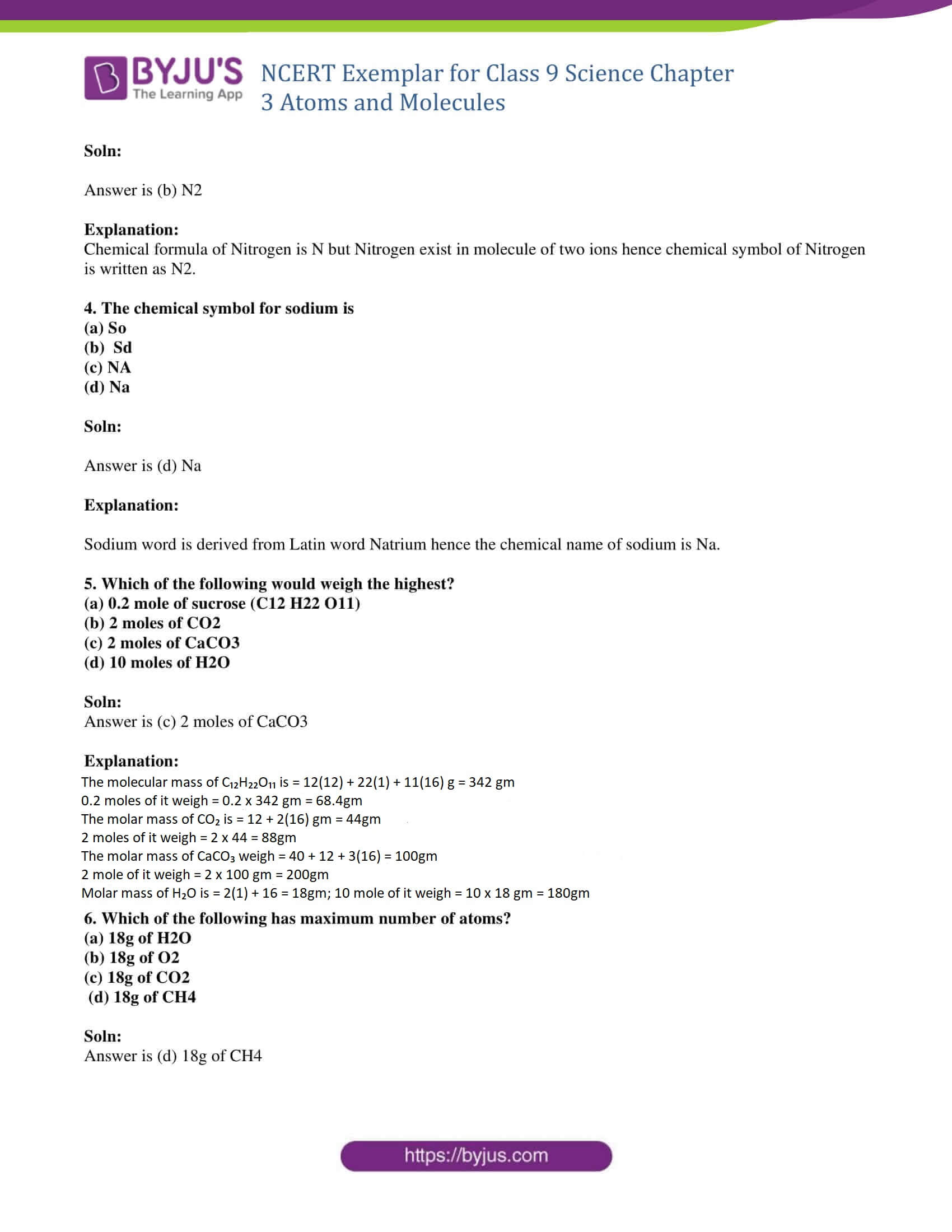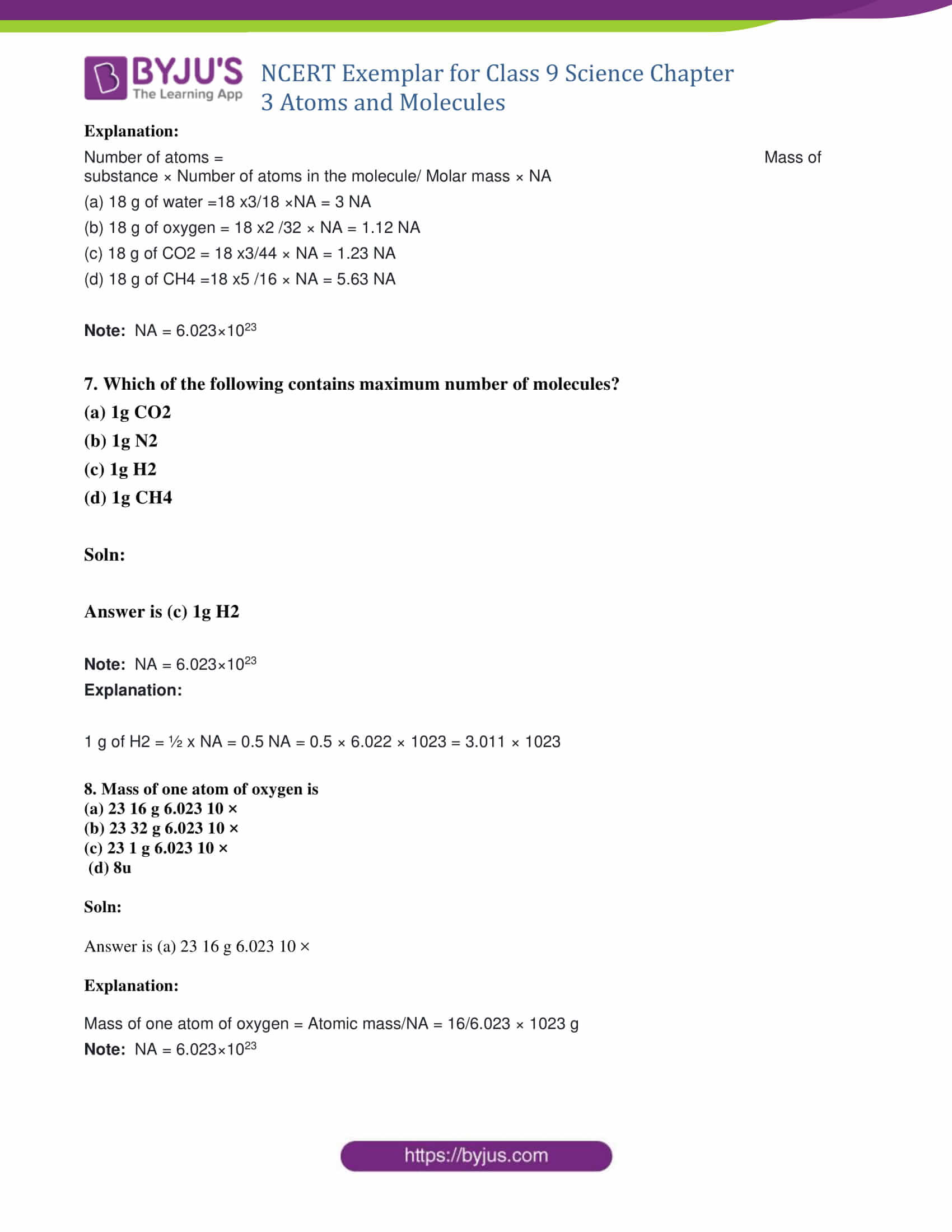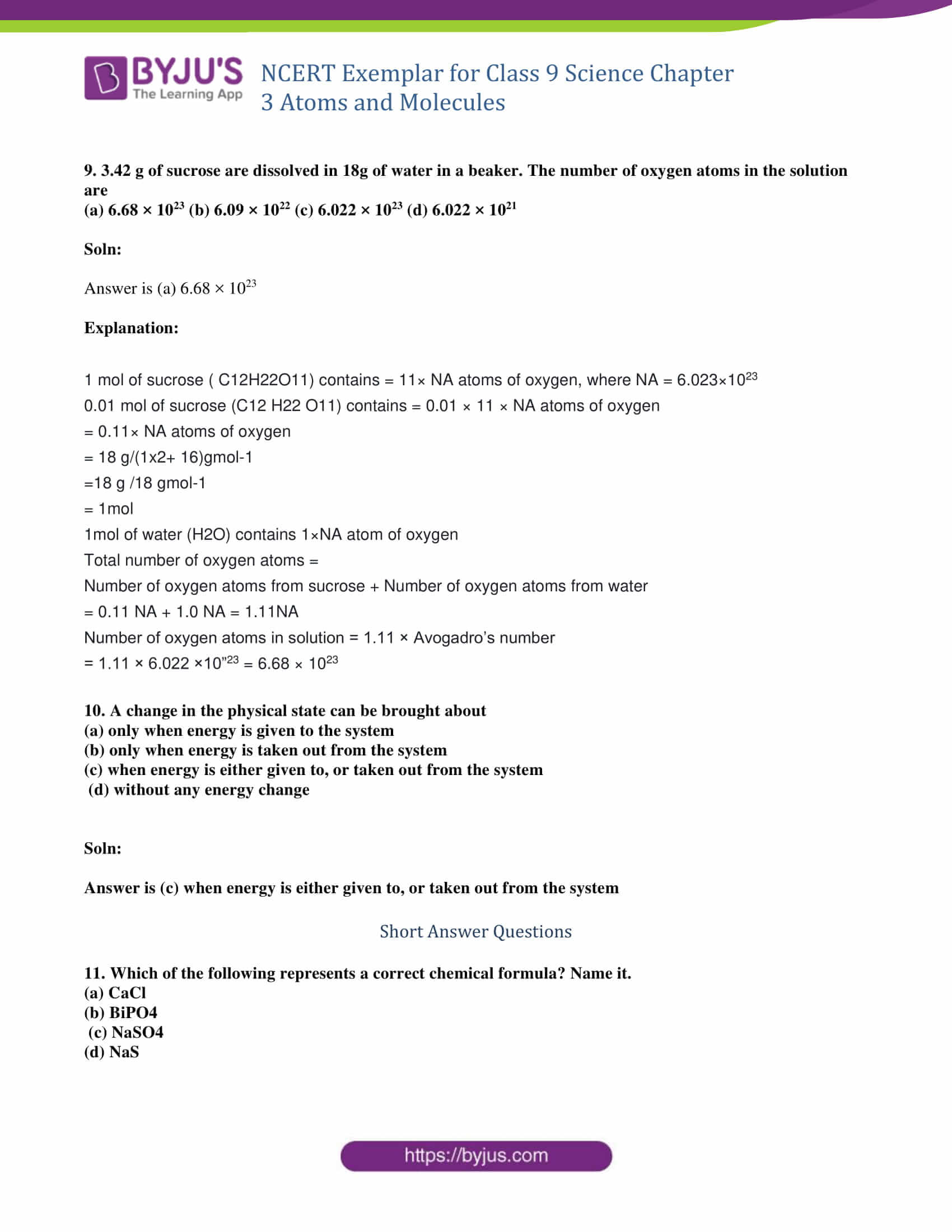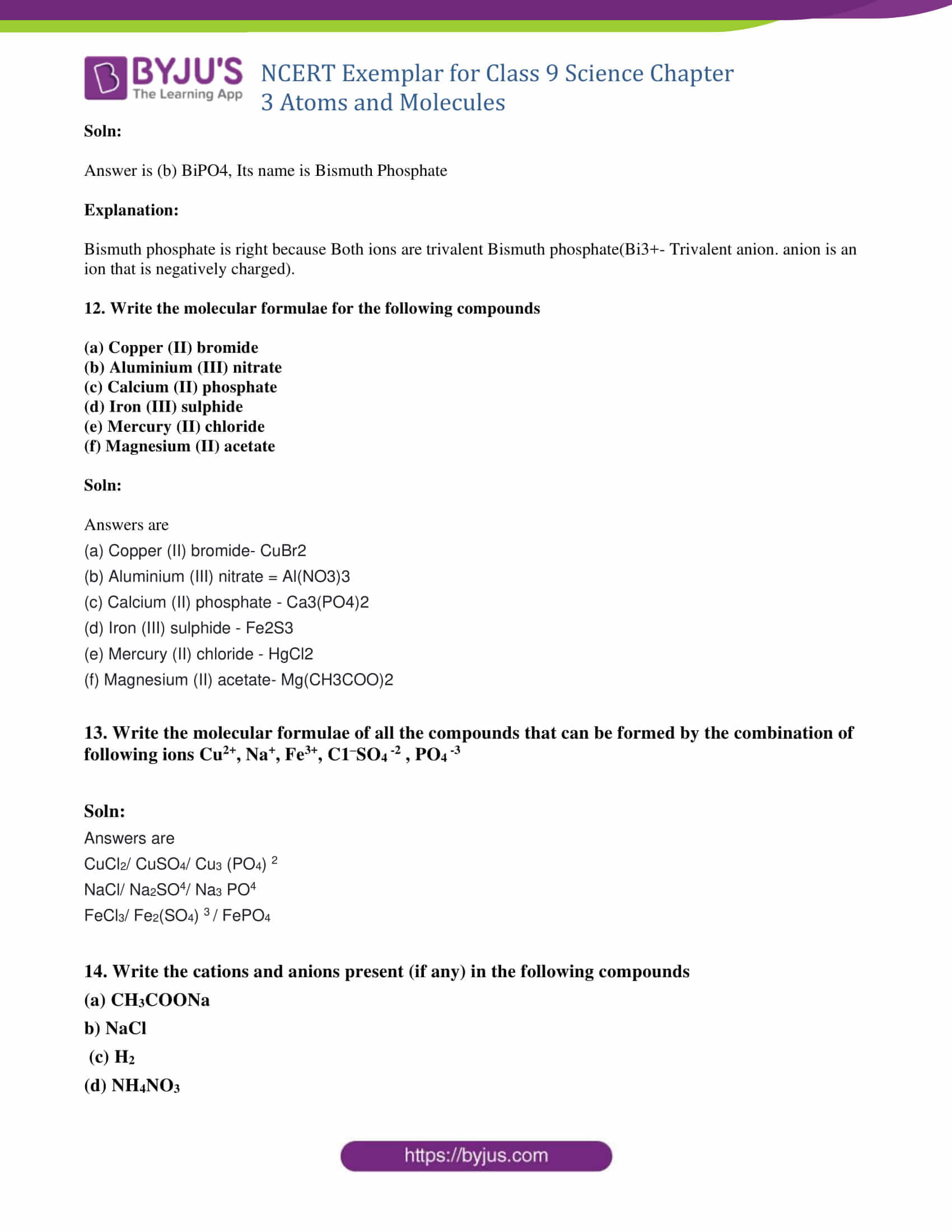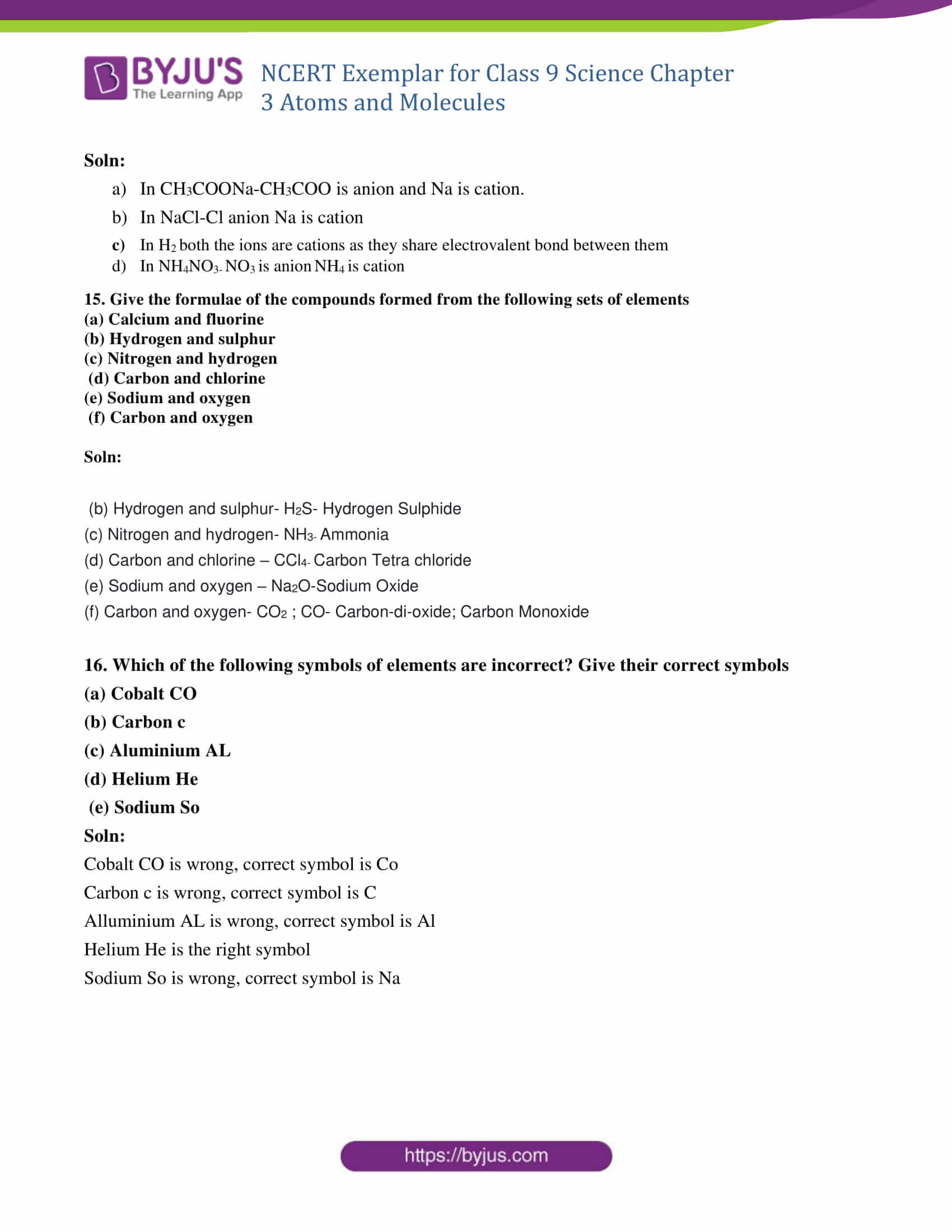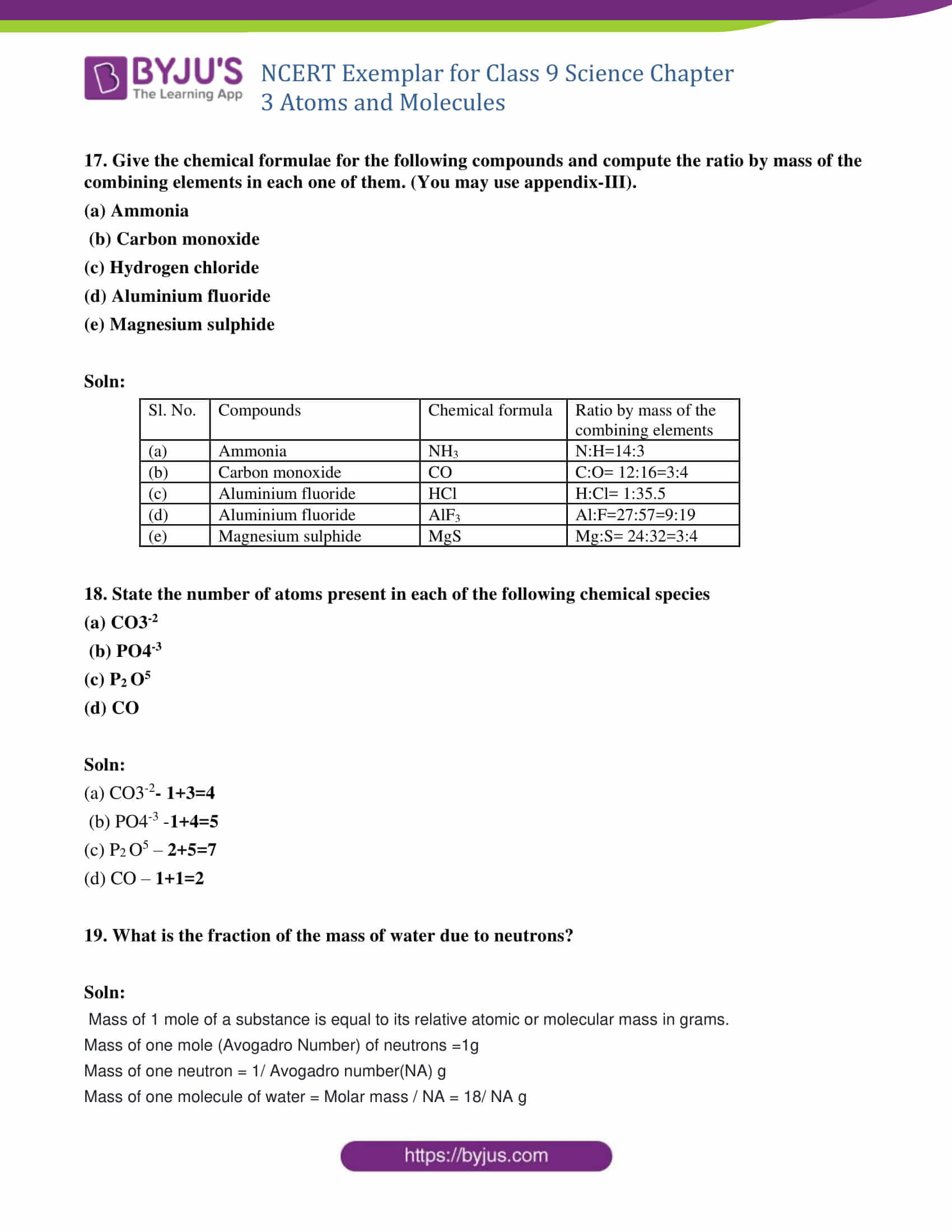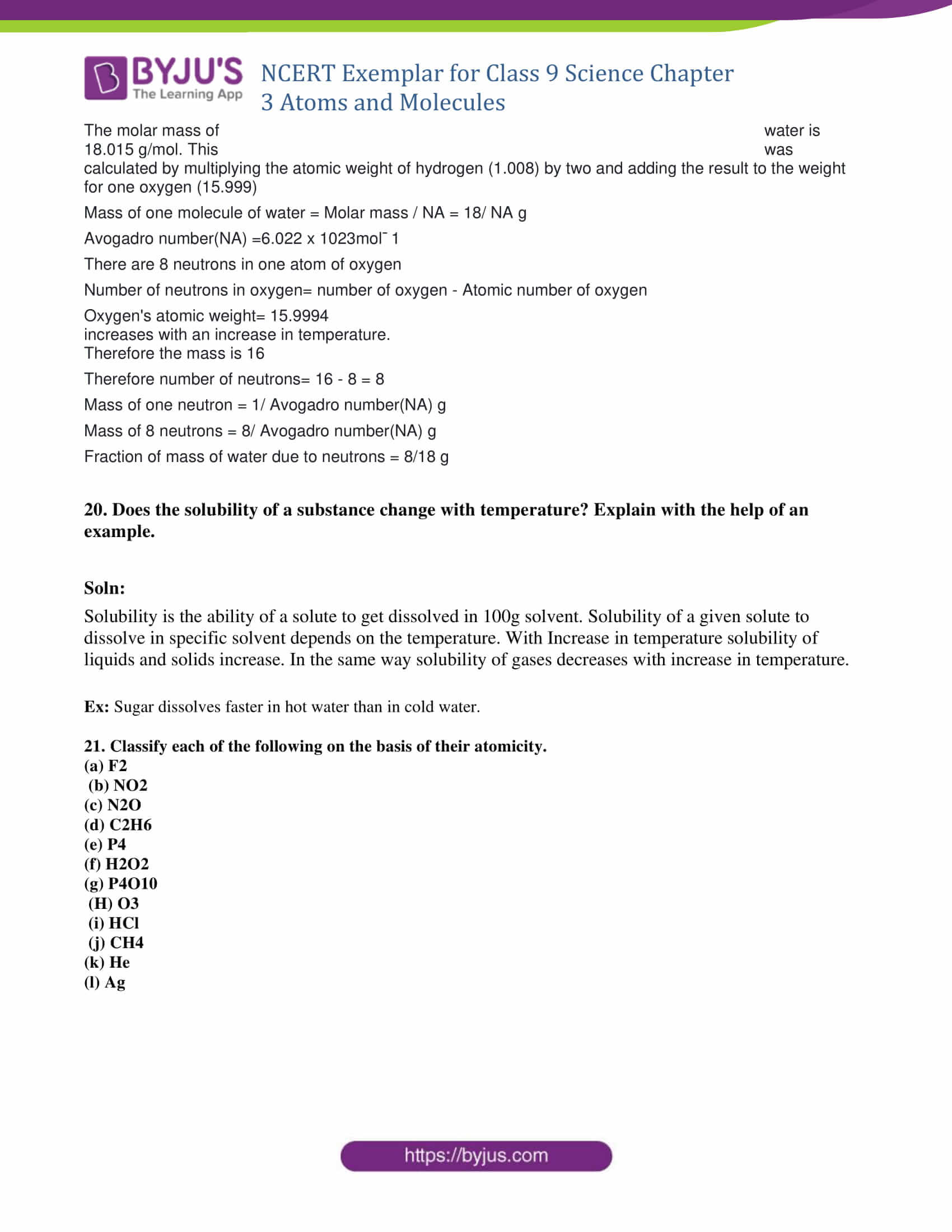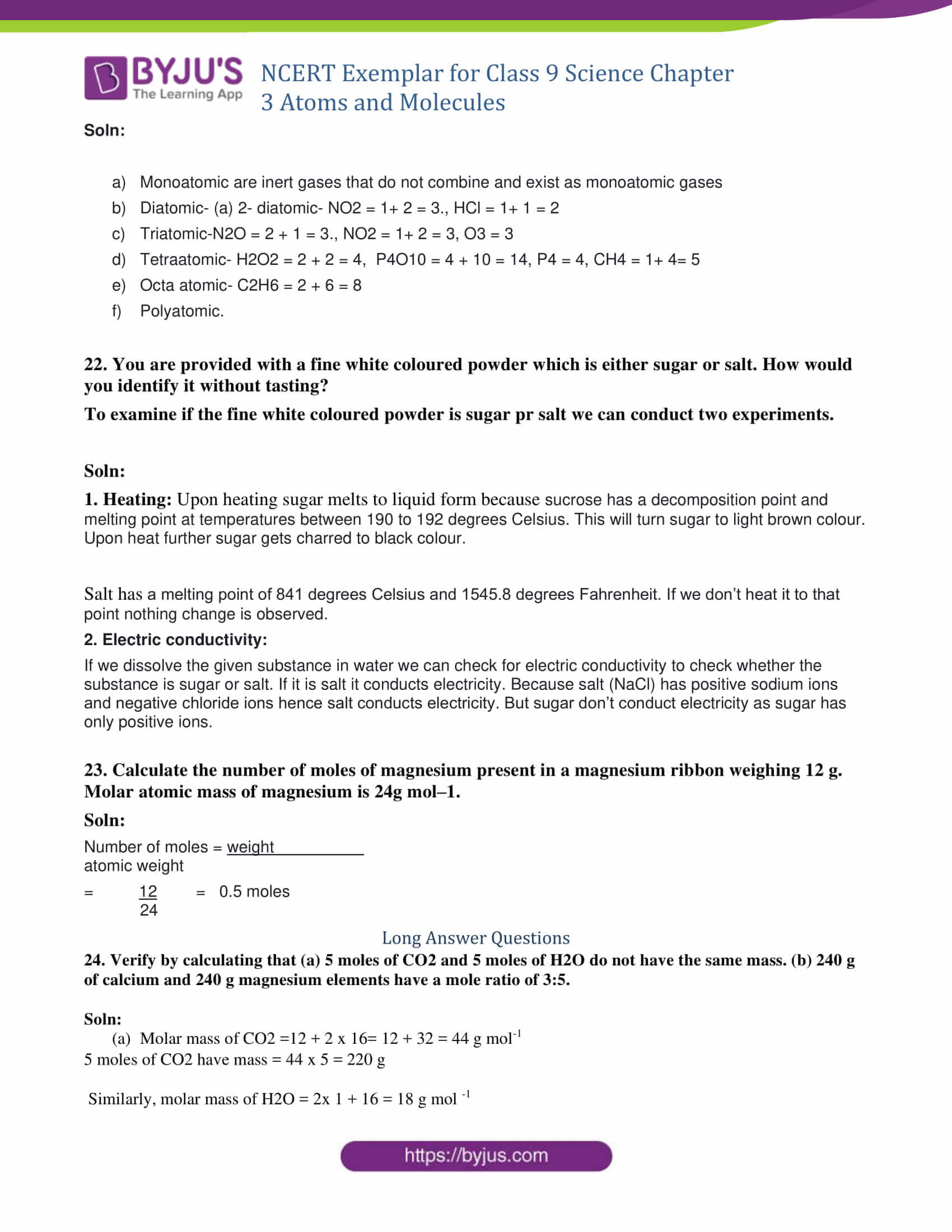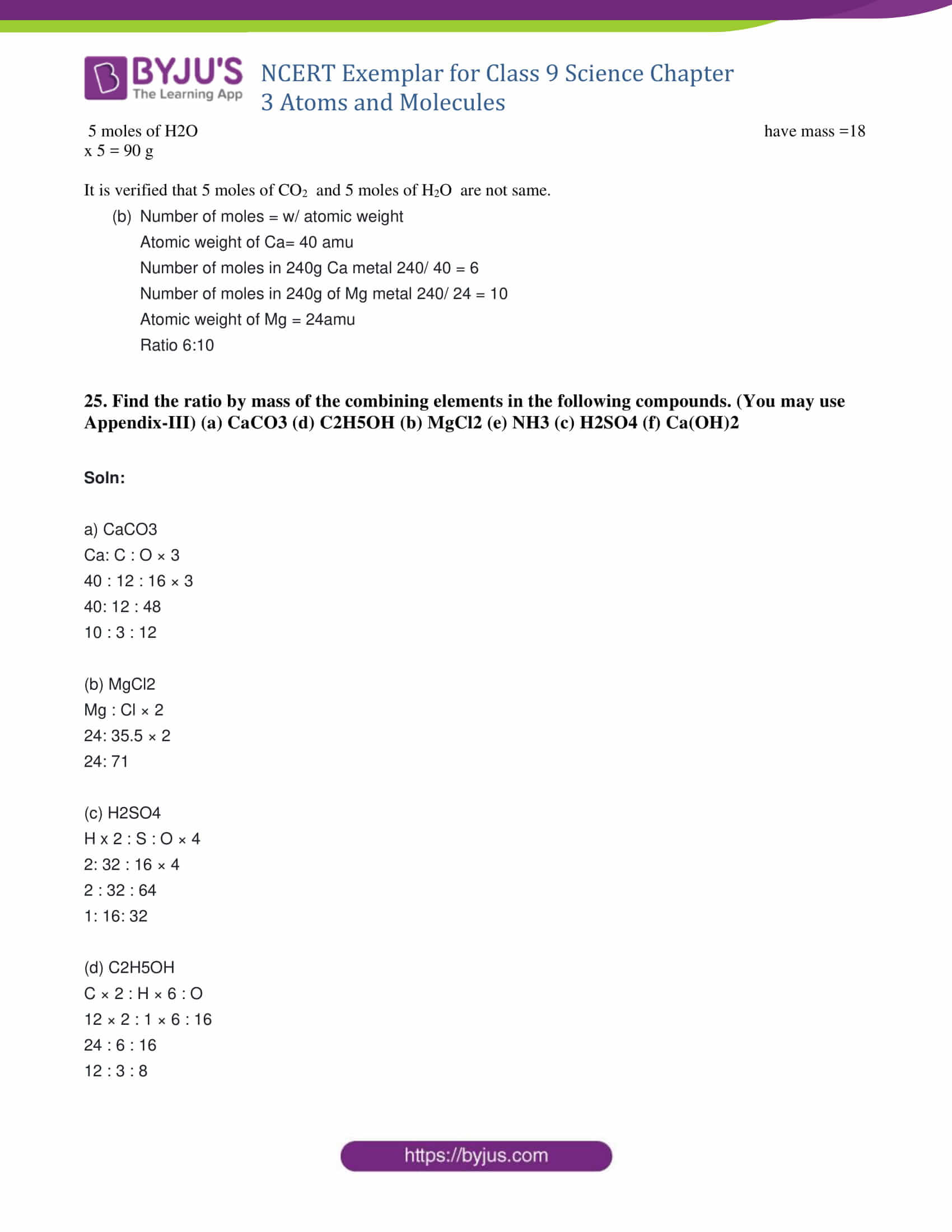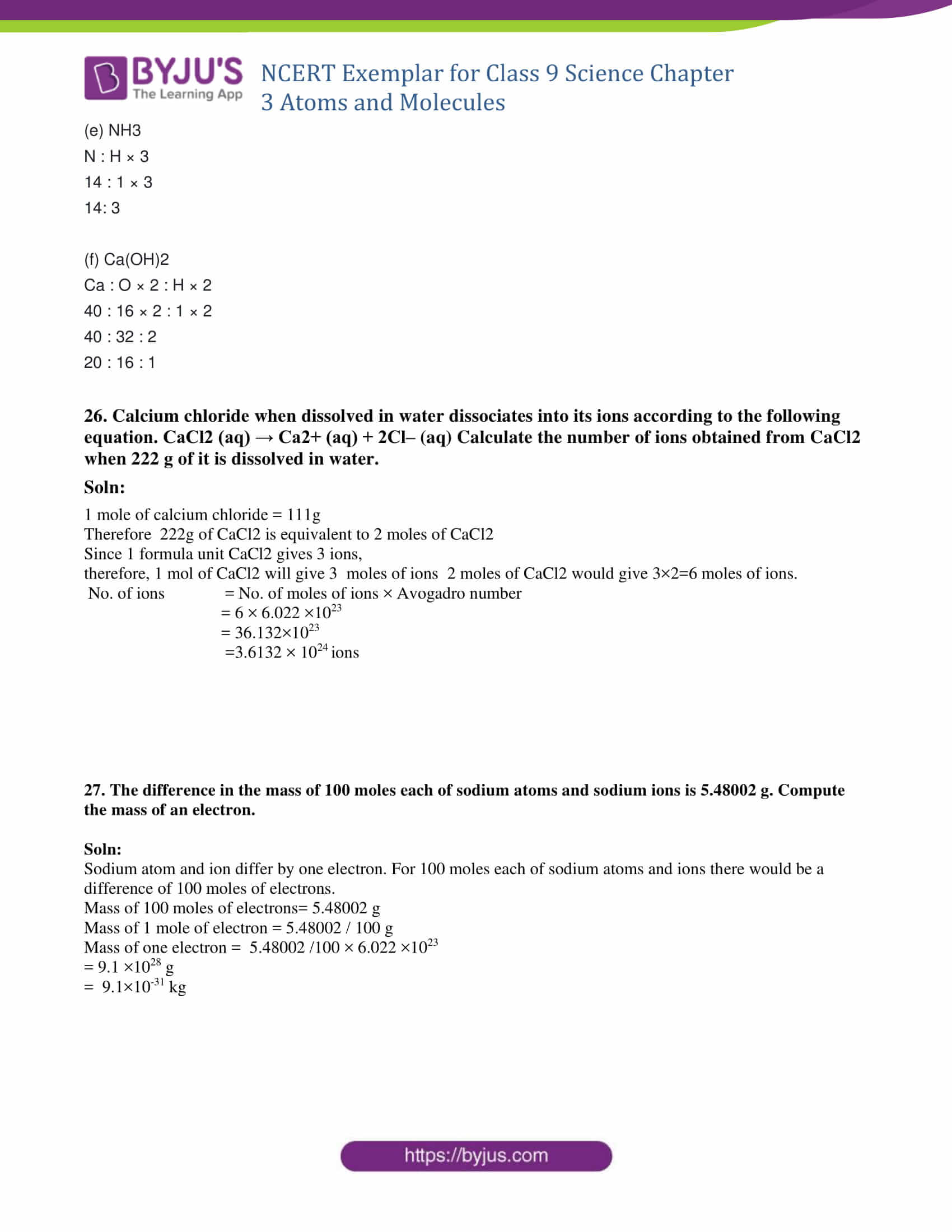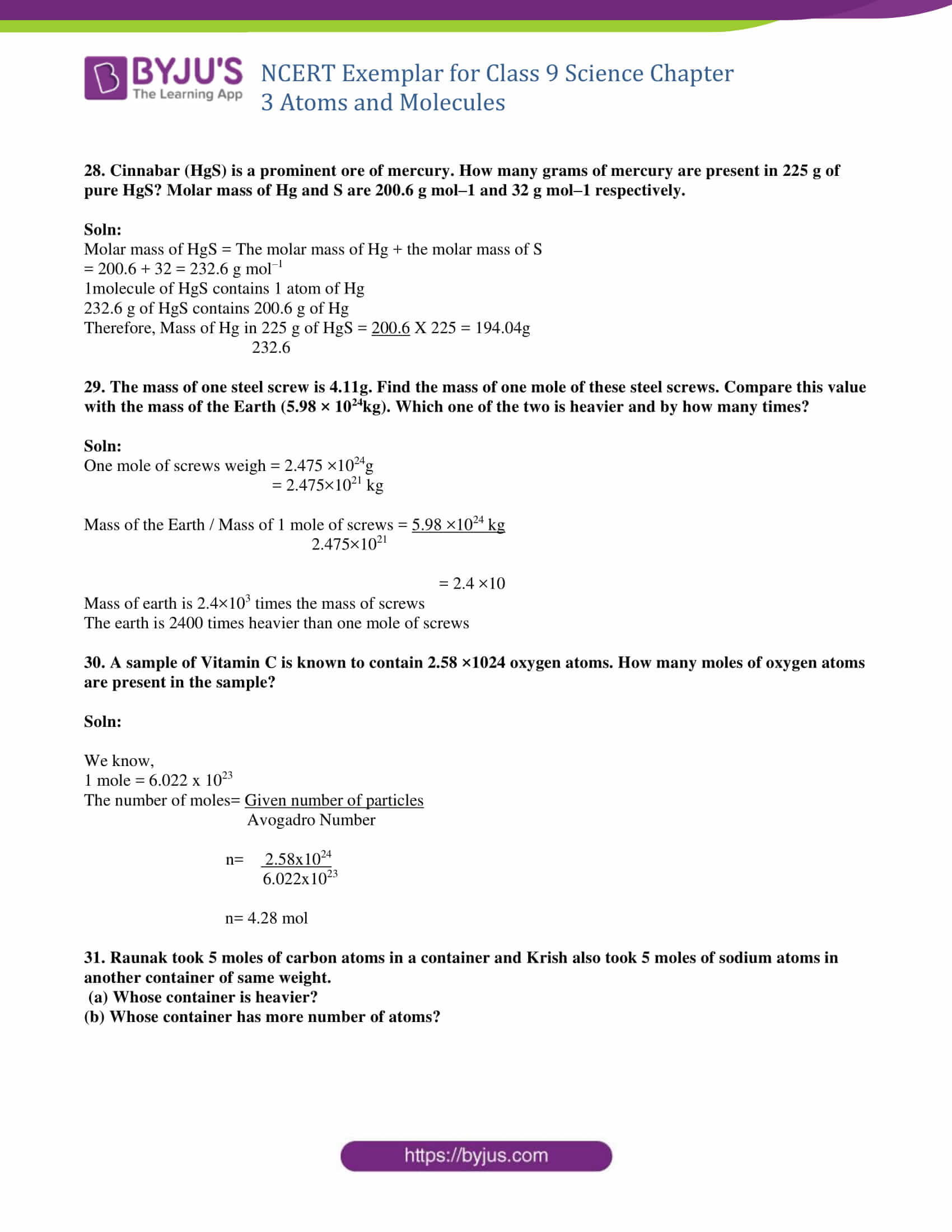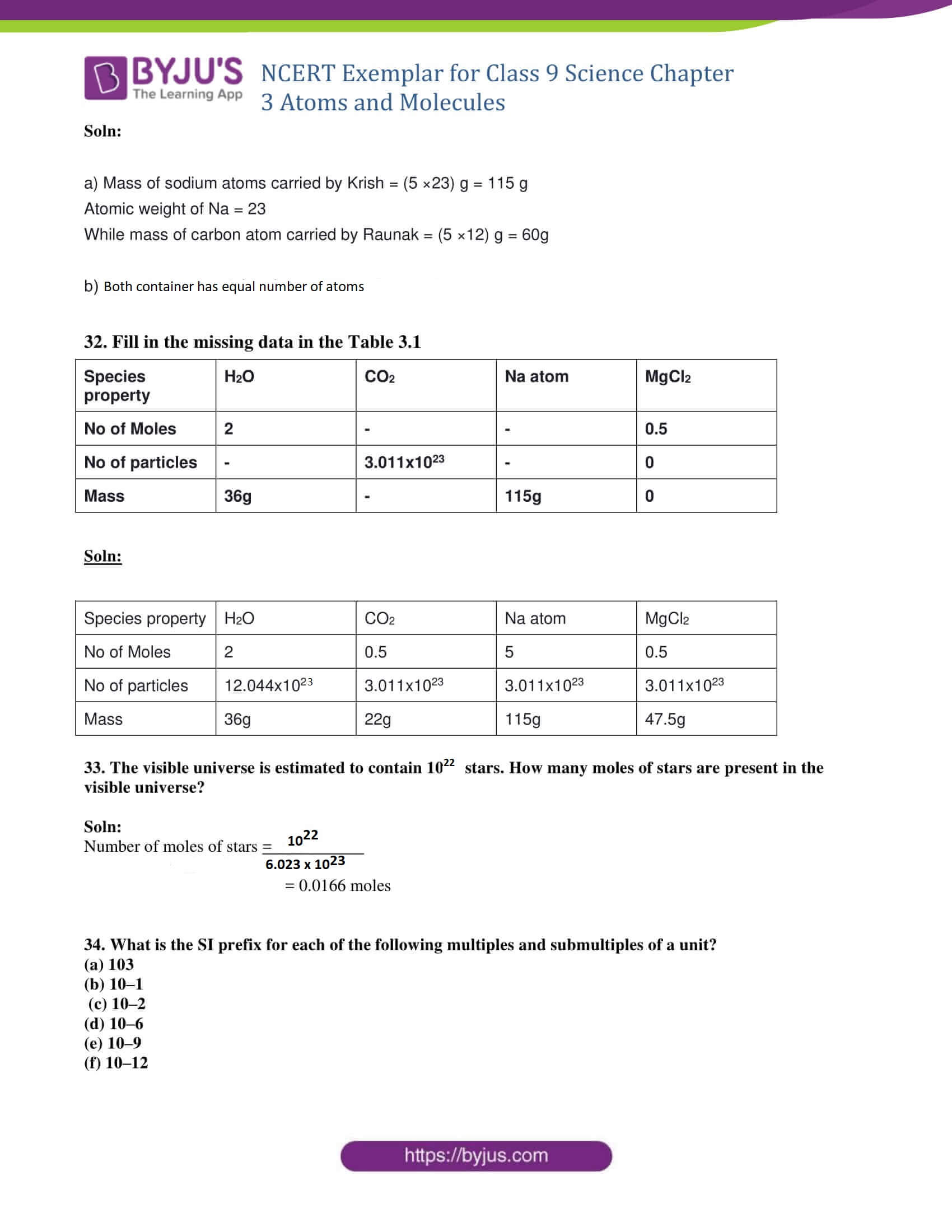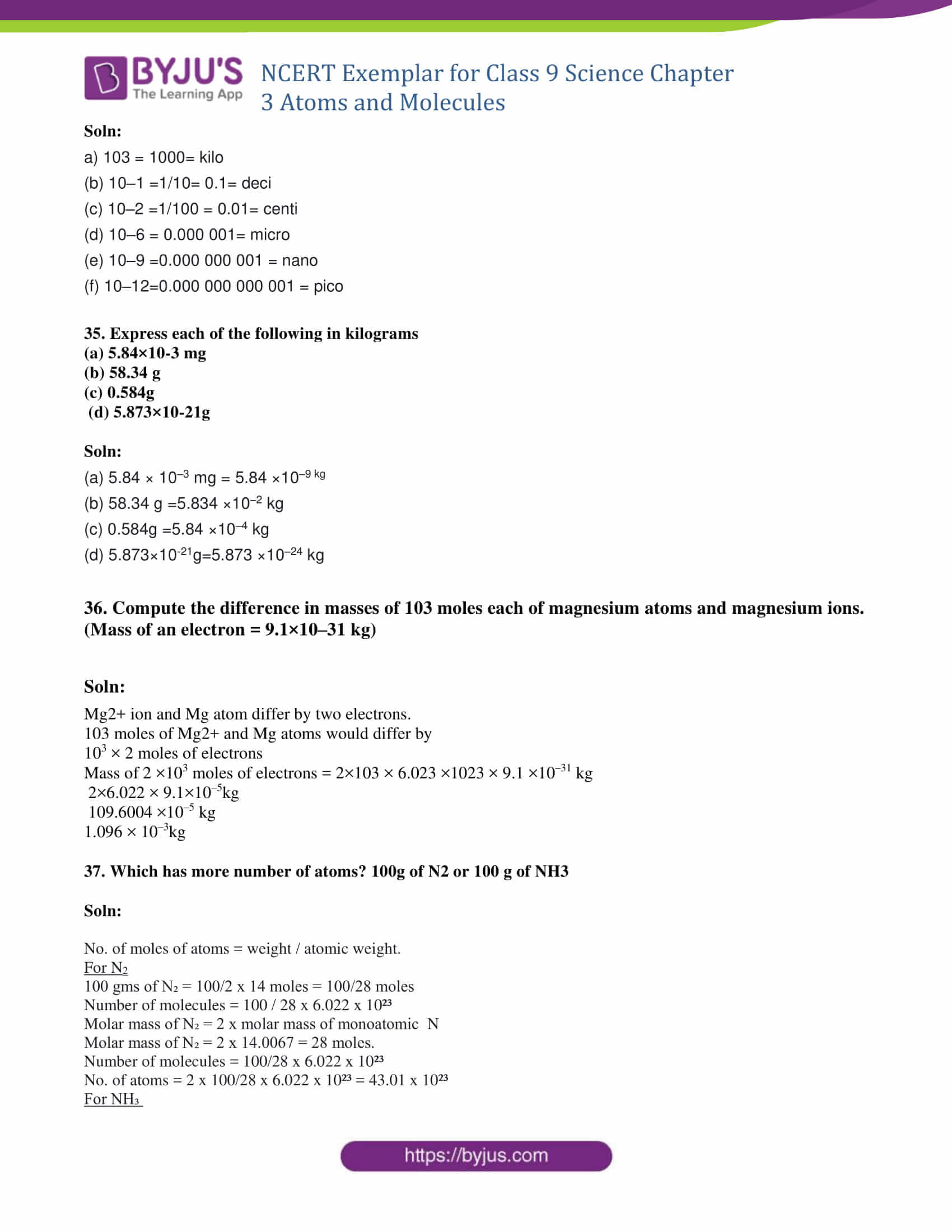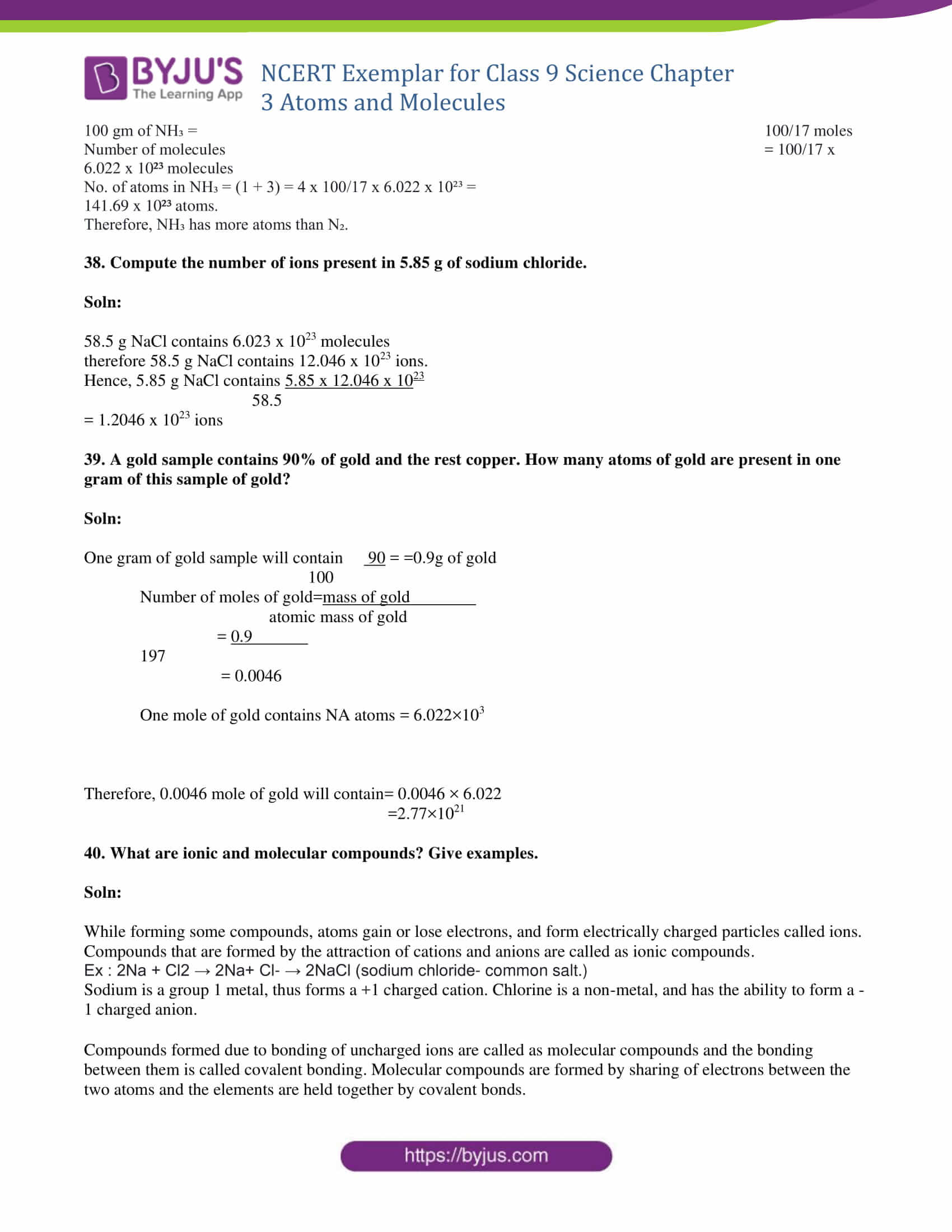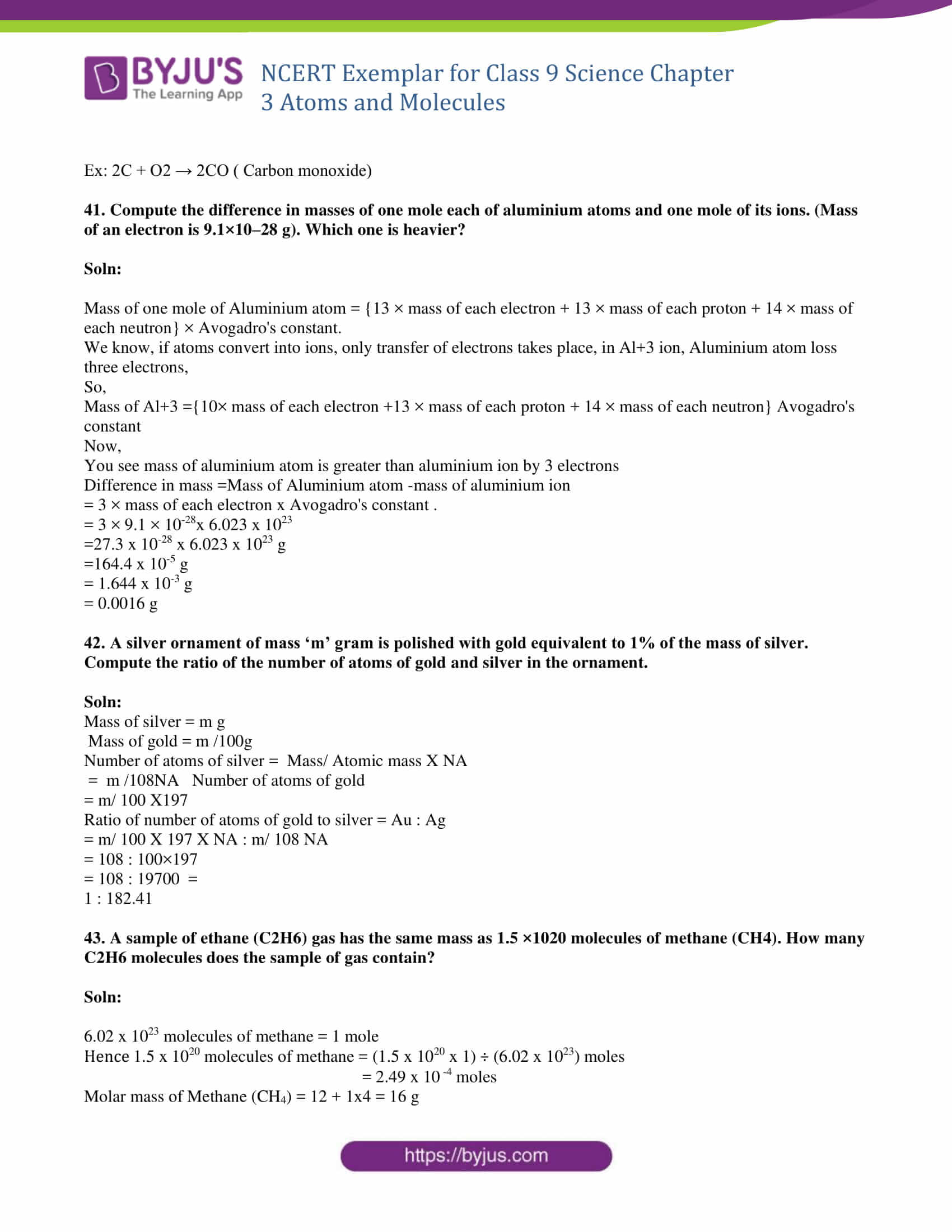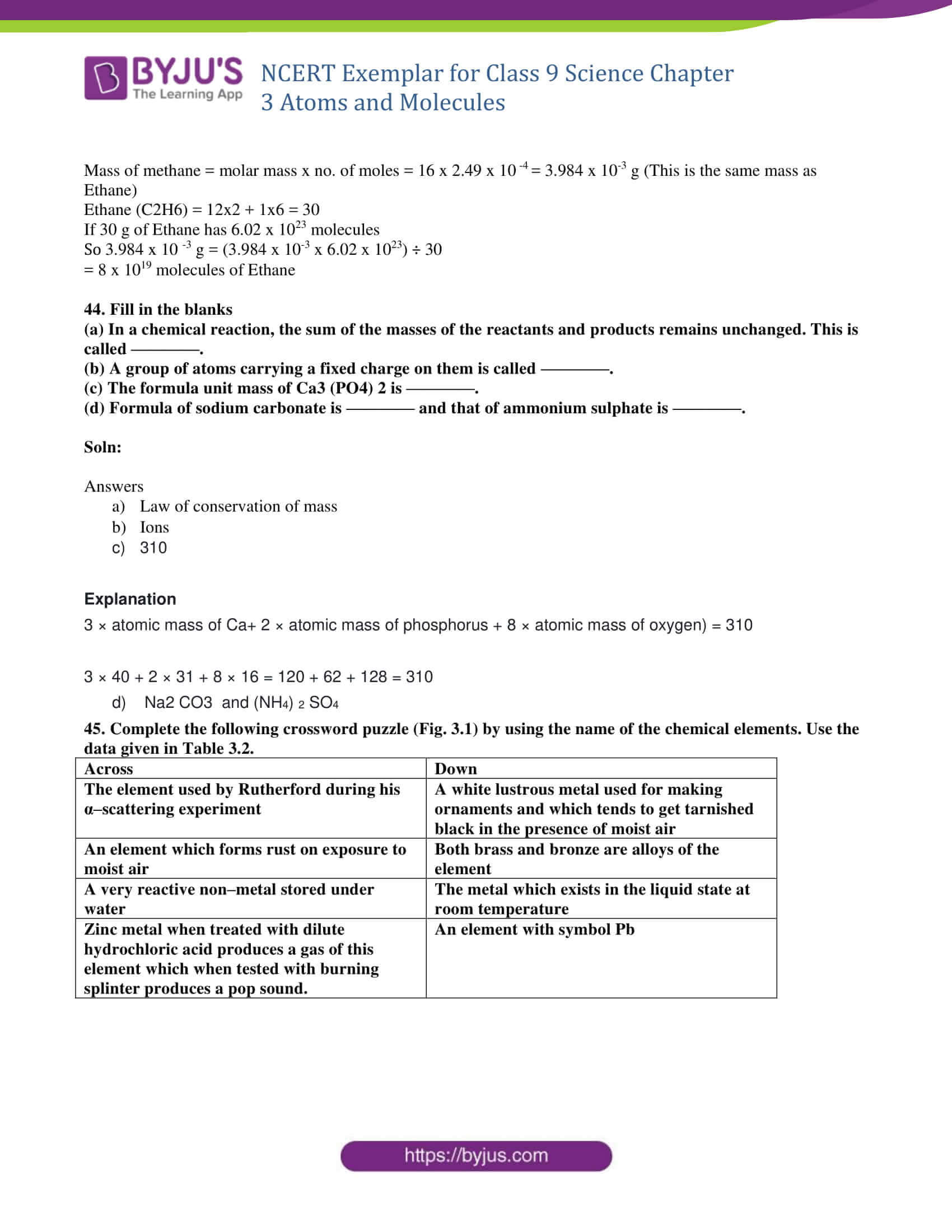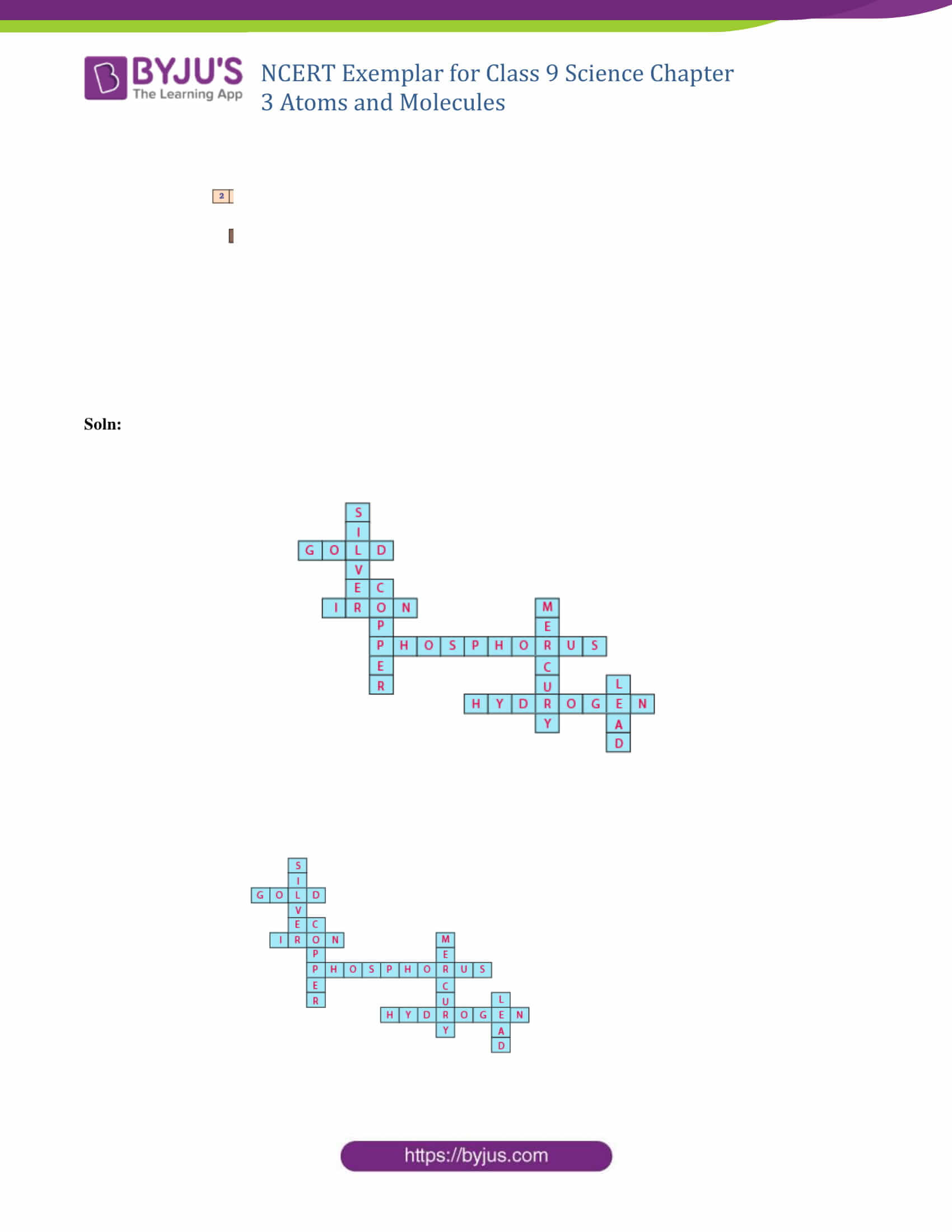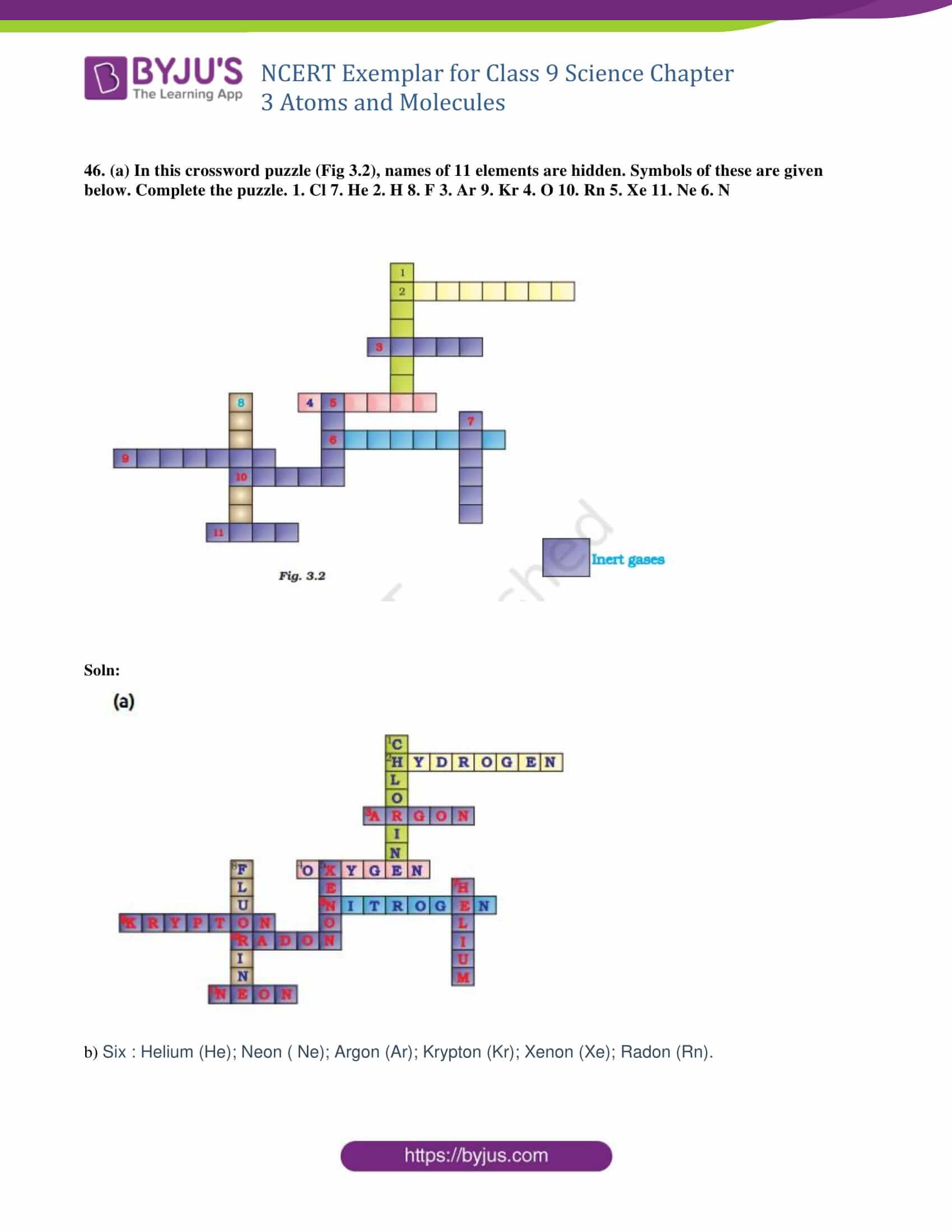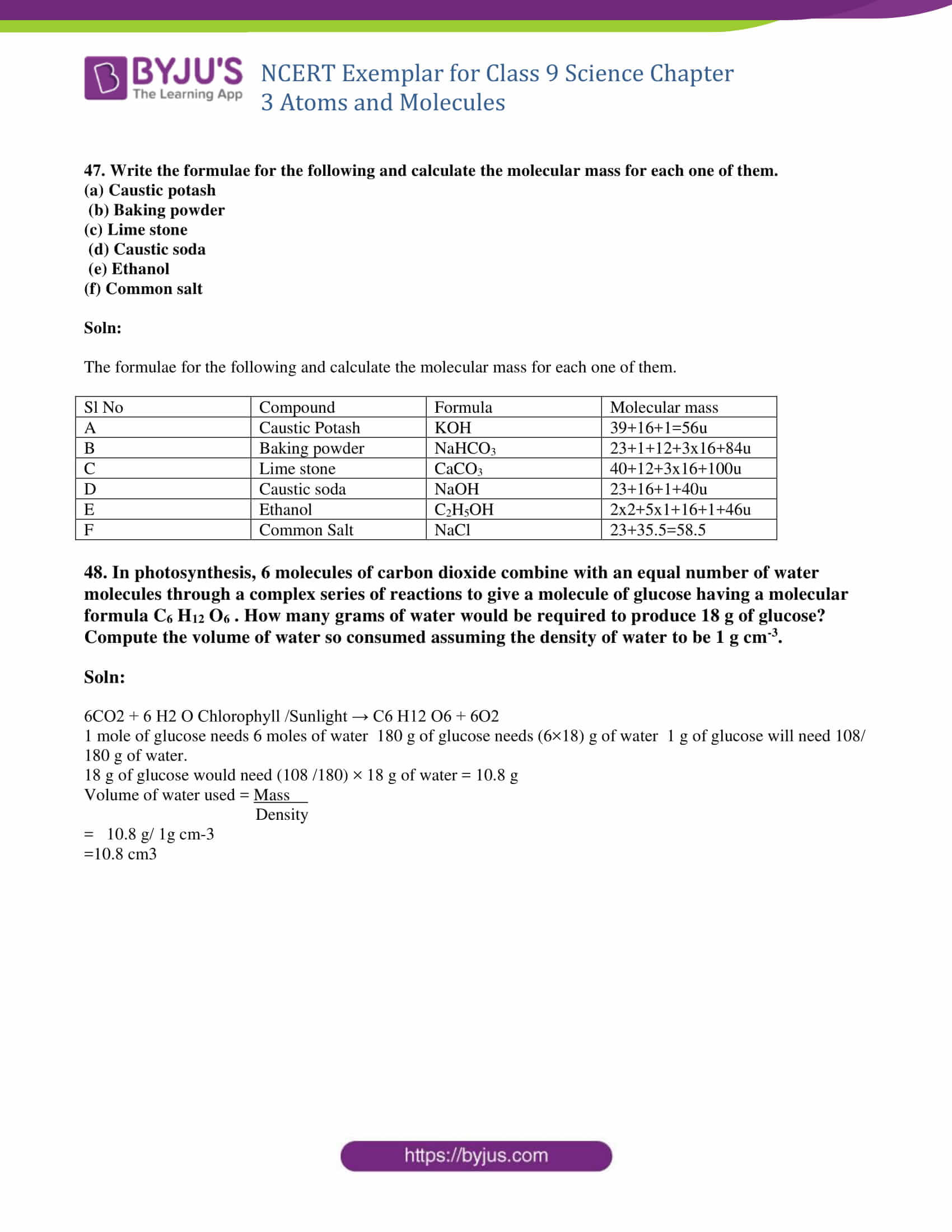### Multiple Choice Questions

1. Which of the following correctly represents 360 g of water?

(i) 2 moles of H20

(ii) 20 moles of water

(iii) 6.022 × 1023 molecules of water

(iv) 1.2044×1025 molecules of water

Choose the option :

(a) (i)

(b) (i) and (iv)

(c) (ii) and (iii)

(d) (ii) and (iv)

Soln:

Answer is (d) (ii) and (iv)

Explanation:

Number of moles = Mass of water / Molar mass of water

Number of moles = 360g / 18g/mol

Number of moles = 20

Number of molecules = 20 x 6.022 x 1023 = 1.2044 x 1025 molecules of water

Thus, option (d) is correct.

2. Which of the following statements is not true about an atom?

(a) Atoms are not able to exist independently

(b) Atoms are the basic units from which molecules and ions are formed

(c) Atoms are always neutral in nature

d) Atoms aggregate in large numbers to form the matter that we can see, feel or touch

Soln:

Answer is (a) Atoms are not able to exist independently

3. The chemical symbol for nitrogen gas is

(a) Ni

(b) N2

(c) N+

(d) N

Soln:

Explanation:

The chemical formula of Nitrogen is N but Nitrogen exist in molecule of two ions hence chemical symbol of Nitrogen gas is written as N2.

4. The chemical symbol for sodium is

(a) So

(b) Sd

(c) NA

(d) Na

Soln:

Explanation:

Sodium word is derived from Latin word Natrium hence the chemical name of sodium is Na.

5. Which of the following would weigh the highest?

(a) 0.2 mole of sucrose (C12 H22 O11)

(b) 2 moles of CO2

(c) 2 moles of CaCO3

(d) 10 moles of H2O

Soln:

Answer is (c) 2 moles of CaCO3

Explanation:

The molecular mass of C₁₂H₂₂O₁₁ is = 12(12) + 22(1) + 11(16) gm = 342 gm

0.2 moles of it weigh = 0.2 x 342 gm = 68.4gm

The molar mass of CO₂ is = 12 + 2(16) gm = 44gm

2 moles of it weigh = 2 x 44 = 88gm

The molar mass of CaCO₃ weigh = 40 + 12 + 3(16) = 100gm

2 mole of it weigh = 2 x 100 gm = 200gm

Molar mass of H₂O is = 2(1) + 16 = 18gm

10 mole of it weigh = 10 x 18 gm = 180gm

6. Which of the following has maximum number of atoms?

(a) 18g of H2O

(b) 18g of O2

(c) 18g of CO2

(d) 18g of CH4

Soln:

Answer is (d) 18g of CH4

Explanation:

Number of atoms = Mass of substance × Number of atoms in the molecule/ Molar mass × NA

(a) 18 g of water =18 x3/18 ×NA = 3 NA

(b) 18 g of oxygen = 18 x2 /32 × NA = 1.12 NA

(c) 18 g of CO2 = 18 x3/44 × NA = 1.23 NA

(d) 18 g of CH4 =18 x5 /16 × NA = 5.63 NA

Note: NA = 6.023×1023

7. Which of the following contains maximum number of molecules?

(a) 1g CO2

(b) 1g N2

(c) 1g H2

(d) 1g CH4

Soln:

Note: NA = 6.023×1023

Explanation:

1 g of H2 = ½ x NA = 0.5 NA = 0.5 × 6.022 × 1023 = 3.011 × 1023

8. Mass of one atom of oxygen is

a) 16/ 6.023 × 1023g

b) 32/ 6.023 × 1023g

c) 3/ 6.023 × 1023g

(d) 8u

Soln:

Answer is (a) 16/ 6.023 × 1023g

Explanation:

Mass of one atom of oxygen = Atomic mass/NA = 16/ 6.023 × 1023g

Note: NA = 6.023×1023

9. 3.42 g of sucrose are dissolved in 18g of water in a beaker. The number of oxygen atoms in the solution are

(a) 6.68 × 1023 (b) 6.09 × 1022 (c) 6.022 × 1023 (d) 6.022 × 1021

Soln:

Answer is (a) 6.68 × 1023

Explanation:

1 mol of sucrose ( C12H22O11) contains = 11× NA atoms of oxygen, where NA = 6.023×1023

0.01 mol of sucrose (C12 H22 O11) contains = 0.01 × 11 × NA atoms of oxygen

= 0.11× NA atoms of oxygen

= 18 g/(1×2+ 16)gmol-1

=18 g /18 gmol-1

= 1mol

1mol of water (H2O) contains 1×NA atom of oxygen

Total number of oxygen atoms =

Number of oxygen atoms from sucrose + Number of oxygen atoms from water

= 0.11 NA + 1.0 NA = 1.11NA

Number of oxygen atoms in solution = 1.11 × Avogadro’s number

= 1.11 × 6.022 ×10”23 = 6.68 × 1023

10. A change in the physical state can be brought about

(a) only when energy is given to the system

(b) only when energy is taken out from the system

(c) when energy is either given to or taken out from the system

(d) without any energy change

Soln:

Answer is (c) when energy is either given to or taken out from the system

11. Which of the following represents a correct chemical formula? Name it.

(a) CaCl

(b) BiPO4

(c) NaSO4

(d) NaS

Soln:

Answer is (b) BiPO4, Its name is Bismuth Phosphate

Explanation:

Bismuth phosphate is right because Both ions are trivalent Bismuth phosphate(Bi3+- Trivalent anion. anion is an ion that is negatively charged).

12. Write the molecular formulae for the following compounds

(a) Copper (II) bromide

(b) Aluminium (III) nitrate

(c) Calcium (II) phosphate

(d) Iron (III) sulphide

(e) Mercury (II) chloride

(f) Magnesium (II) acetate

Soln:

(a) Copper (II) bromide = CuBr2

(b) Aluminium (III) nitrate = Al(NO3)3

(c) Calcium (II) phosphate = Ca3(PO4)2

(d) Iron (III) sulphide = Fe2S3

(e) Mercury (II) chloride = HgCl2

(f) Magnesium (II) acetate = Mg(CH3COO)2

13. Write the molecular formulae of all the compounds that can be formed by the combination of following ions Cu2+, Na+, Fe3+, Cl–  SO4 -2, PO4 -3

Soln:

CuCl2/ CuSO4/ Cu3(PO4) 2

NaCl/ Na2SO4/ Na3PO4

FeCl3/ Fe2(SO4) 3 / FePO4

14. Write the cations and anions present (if any) in the following compounds

(a) CH3COONa

b) NaCl

(c) H2

(d) NH4NO3

Soln:

1. In CH3COONa-CH3COO is anion and Na is cation.
2. In NaCl-Cl anion Na is cation
3. In H2both the ions are cations as they share electrovalent bond between them
4. In NH4NO3- NO3 is anion NH4 is cation

15. Give the formulae of the compounds formed from the following sets of elements

(a) Calcium and fluorine

(b) Hydrogen and sulphur

(c) Nitrogen and hydrogen

(d) Carbon and chlorine

(e) Sodium and oxygen

(f) Carbon and oxygen

Soln:

(a) Calcium and fluoride – Calcium Fluoride (CaF2)

(b) Hydrogen and sulphur- H2S- Hydrogen Sulphide

(c) Nitrogen and hydrogen- NH3- Ammonia

(d) Carbon and chlorine – CCl4- Carbon Tetrachloride

(e) Sodium and oxygen – Na2O-Sodium Oxide

(f) Carbon and oxygen- CO2 ; CO- Carbon-di-oxide; Carbon Monoxide

16. Which of the following symbols of elements are incorrect? Give their correct symbols

(a) Cobalt CO

(b) Carbon c

(c) Aluminium AL

(d) Helium He

(e) Sodium So

Soln:

Cobalt CO is wrong, correct symbol is Co

Carbon c is wrong, correct symbol is C

Aluminium AL is wrong, correct symbol is Al

Helium He is the right symbol

Sodium So is wrong, correct symbol is Na

17. Give the chemical formulae for the following compounds and compute the ratio by mass of the combining elements in each one of them. (You may use appendix-III).

(a) Ammonia

(b) Carbon monoxide

(c) Hydrogen chloride

(d) Aluminium fluoride

(e) Magnesium sulphide

Soln:

 S. No. Compounds Chemical formula Ratio by mass of the combining elements (a) Ammonia NH3 N:H=14:3 (b) Carbon monoxide CO C:O= 12:16=3:4 (c) Aluminium fluoride HCl H:Cl= 1:35.5 (d) Aluminium fluoride AlF3 Al:F=27:57=9:19 (e) Magnesium sulphide MgS Mg:S= 24:32=3:4

18. State the number of atoms present in each of the following chemical species

(a) CO3-2

(b) PO4-3

(c) P2 O5

(d) CO

Soln:

(a) CO3-2  1+3=4

(b) PO4-3 – 1+4=5

(c) P2O5 –  2+5=7

(d) CO –  1+1=2

19. What is the fraction of the mass of water due to neutrons?

Soln:

Mass of 1 mole of a substance is equal to its relative atomic or molecular mass in grams.

Mass of one mole (Avogadro Number) of neutrons =1g

Mass of one neutron = 1/ Avogadro number(NA) g

Mass of one molecule of water = Molar mass / NA = 18/ NA g

The molar mass of water is 18.015 g/mol. This was calculated by multiplying the atomic weight of hydrogen (1.008) by two and adding the result to the weight for one oxygen (15.999)

Mass of one molecule of water = Molar mass / NA = 18/ NA g

There are 8 neutrons in one atom of oxygen

Number of neutrons in oxygen= number of oxygen – Atomic number of oxygen

Oxygen’s atomic weight= 15.9994

increases with an increase in temperature.

Therefore the mass is 16

Therefore number of neutrons= 16 – 8 = 8

Mass of one neutron = 1/ Avogadro number(NA) g

Mass of 8 neutrons = 8/ Avogadro number(NA) g

Fraction of mass of water due to neutrons = 8/18 g

20. Does the solubility of a substance change with temperature? Explain with the help of an example.

Soln:

Solubility is the ability of a solute to get dissolved in 100g solvent. Solubility of a given solute to dissolve in specific solvent depends on the temperature. With an increase in temperature solubility of liquids and solids increase. In the same way solubility of gases decreases with increase in temperature.

Ex: Sugar dissolves faster in hot water than in cold water.

21. Classify each of the following on the basis of their atomicity.

(a) F2

(b) NO2

(c) N2O

(d) C2H6

(e) P4

(f) H2O2

(g) P4O10

(H) O3

(i) HCl

(j) CH4

(k) He

(l) Ag

Soln:

a. Diatomic (2 atoms)

b. Triatomic (3 atoms)

c. Triatomic (3 atoms)

d. Polyatomic (8 atoms)

e. Polyatomic (4 atoms)

f. Polyatomic (4 atoms)

g. Polyatomic (14 atoms)

h. Triatomic (3 atoms)

i. Diatomic (2 atoms)

j. Polyatomic (5 atoms)

k. Monoatomic (1 atom)

l. Monoatomic (1 atom)

22. You are provided with a fine white coloured powder which is either sugar or salt. How would you identify it without tasting?

To examine if the fine white coloured powder is sugar pr salt we can conduct two experiments.

Soln:

Heating:

Upon heating sugar melts to liquid form because sucrose has a decomposition point and melting point at temperatures between 190 to 192 degrees Celsius. This will turn sugar to light brown colour. On heating further, sugar gets charred to black colour.

Salt has a melting point of 841 degrees Celsius and 1545.8 degrees Fahrenheit. If we don’t heat it to that point no change is observed.

Electrical conductivity:

If we dissolve the given substance in water we can check for electrical conductivity to check whether the substance is sugar or salt. If it is salt it conducts electricity. Because salt (NaCl) has positive sodium ions and negative chloride ions hence salt conducts electricity. But sugar doesn’t conduct electricity as sugar has only positive ions.

23. Calculate the number of moles of magnesium present in a magnesium ribbon weighing 12 g. Molar atomic mass of magnesium is 24 g mol–1.

Soln:

Number of moles = weight atomic weight

= 12/24 = 0.5 moles

24. Verify by calculating that (a) 5 moles of CO2 and 5 moles of H2O do not have the same mass. (b) 240 g of calcium and 240 g magnesium elements have a mole ratio of 3:5.

Soln:

a) Molar mass of CO2 = 12 + 2 x 16 = 12 + 32 = 44 g mol-1

5 moles of CO2 have mass = 44 x 5 = 220 g

Similarly, molar mass of H2O = 2x 1 + 16 = 18 g mol -1

5 moles of H2O have mass =18 x 5 = 90 g

It is verified that 5 moles of CO2 and 5 moles of H2O are not same.

b) Number of moles = w/atomic weight

Atomic weight of Ca= 40 amu

Number of moles in 240g Ca metal 240/ 40 = 6

Number of moles in 240g of Mg metal 240/ 24 = 10

Atomic weight of Mg = 24amu

Ratio 6:10

25. Find the ratio by mass of the combining elements in the following compounds. (You may use Appendix-III) (a) CaCO3 (d) C2H5OH (b) MgCl2 (e) NH3 (c) H2SO4 (f) Ca(OH)2

Soln:

a) CaCO3

Ca: C : O × 3

40 : 12 : 16 × 3

40: 12 : 48

10 : 3 : 12

(b) MgCl2

Mg : Cl × 2

24: 35.5 × 2

24: 71

(c) H2SO4

H x 2 : S : O × 4

2: 32 : 16 × 4

2 : 32 : 64

1: 16: 32

(d) C2H5OH

C × 2 : H × 6 : O

12 × 2 : 1 × 6 : 16

24 : 6 : 16

12 : 3 : 8

(e) NH3

N : H × 3

14 : 1 × 3

14: 3

(f) Ca(OH)2

Ca : O × 2 : H × 2

40 : 16 × 2 : 1 × 2

40 : 32 : 2

20 : 16 : 1

26. Calcium chloride when dissolved in water dissociates into its ions according to the following equation. CaCl2 (aq) → Ca2+ (aq) + 2Cl– (aq) Calculate the number of ions obtained from CaCl2 when 222 g of it is dissolved in water.

Soln:

1 mole of calcium chloride = 111g

Therefore 222g of CaCl2 is equivalent to 2 moles of CaCl2

Since 1 formula unit CaCl2 gives 3 ions,

therefore, 1 mol of CaCl2 will give 3  moles of ions  2 moles of CaCl2 would give 3×2=6 moles of ions.

No. of ions = No. of moles of ions × Avogadro number

= 6 × 6.022 ×1023

= 36.132×1023

= 3.6132 × 1024 ions

27. The difference in the mass of 100 moles each of sodium atoms and sodium ions is 5.48002 g. Compute the mass of an electron.

Soln:

Sodium atom and ion differ by one electron. For 100 moles each of sodium atoms and ions there would be a difference of 100 moles of electrons.

Mass of 100 moles of electrons= 5.48002 g

Mass of 1 mole of electron = 5.48002 / 100 g

Mass of one electron =  5.48002 /100 × 6.022 ×1023

= 9.1 ×1028 g

=  9.1×10-31 kg

28. Cinnabar (HgS) is a prominent ore of mercury. How many grams of mercury are present in 225 g of pure HgS? Molar mass of Hg and S are 200.6 g mol–1 and 32 g mol–1 respectively.

Soln:

The molar mass of HgS = The molar mass of Hg + the molar mass of S

= 200.6 + 32 = 232.6 g mol–1

1molecule of HgS contains 1 atom of Hg

232.6 g of HgS contains 200.6 g of Hg

Therefore, Mass of Hg in 225 g of HgS = 200.6 X 225 /232.6 = 194.04g

29. The mass of one steel screw is 4.11g. Find the mass of one mole of these steel screws. Compare this value with the mass of the Earth (5.98 × 1024kg). Which one of the two is heavier and by how many times?

Soln:

One mole of screws weigh = 2.475 ×1024g

= 2.475×1021 kg

Mass of the Earth / Mass of 1 mole of screws = 5.98 ×1024 kg 2.475×1021

= 2.4 ×10

Mass of earth is 2.4×103 times the mass of screws

The earth is 2400 times heavier than one mole of screws

30. A sample of Vitamin C is known to contain 2.58 ×1024 oxygen atoms. How many moles of oxygen atoms are present in the sample?

Soln:

We know,

1 mole = 6.022 x 1023

The number of moles= Given number of particles / Avogadro Number

n= 2.58×1024 /6.022×1023

n= 4.28 mol

31. Raunak took 5 moles of carbon atoms in a container and Krish also took 5 moles of sodium atoms in another container of same weight.

(a) Whose container is heavier?

(b) Whose container has more number of atoms?

Soln:

a) Mass of sodium atoms carried by Krish = (5 ×23) g = 115 g

The atomic weight of Na = 23

While the mass of carbon atom carried by Raunak = (5 ×12) g = 60g

b) Both container has an equal number of atoms

32. Fill in the missing data in the Table 3.1

 Species property H2O CO2 Na atom MgCl2 No of Moles 2 – – 0.5 No of particles – 3.011×1023 – 0 Mass 36g – 115g 0

Soln:

 Species property H2O CO2 Na atom MgCl2 No of Moles 2 0.5 5 0.5 No of particles 12.044×1023 3.011×1023 3.011×1023 3.011×1023 Mass 36g 22g 115g 47.5g

33. The visible universe is estimated to contain 1022 stars. How many moles are present in the visible universe?

Sol :

Number of moles of stars = 1022 / 6.023 × 1023

Number of moles of stars = 0.0166

34. What is the SI prefix for each of the following multiples and submultiples of a unit?

(a) 103

(b) 10–1

(c) 10–2

(d) 10–6

(e) 10–9

(f) 10–12

Soln:

a) 103 = 1000= kilo

(b) 10–1 =1/10= 0.1= deci

(c) 10–2 =1/100 = 0.01= centi

(d) 10–6 = 0.000 001= micro

(e) 10–9 =0.000 000 001 = nano

(f) 10–12 =0.000 000 000 001 = pico

35. Express each of the following in kilograms

(a) 5.84×10-3 mg

(b) 58.34 g

(c) 0.584g

(d) 5.873×10-21g

Soln:

(a) 5.84 × 10–3 mg = 5.84 ×10–9 kg

(b) 58.34 g =5.834 ×10–2 kg

(c) 0.584g =5.84 ×10–4 kg

(d) 5.873×10-21g=5.873 ×10–24 kg

36. Compute the difference in masses of 103 moles each of magnesium atoms and magnesium ions. (Mass of an electron = 9.1×10–31 kg)

Soln:

Mg2+ ion and Mg atom differ by two electrons.

103 moles of Mg2+ and Mg atoms would differ by

103 × 2 moles of electrons

Mass of 2 ×103 moles of electrons = 2×103 × 6.023 ×1023 × 9.1 ×10–31 kg

2×6.022 × 9.1×10–5kg

109.6004 ×10–5 kg

1.096 × 10–3kg

37. Which has more number of atoms? 100g of N2 or 100 g of NH3

Soln:

No. of moles of atoms = weight / atomic weight.

For N2

100 gms of N2 = 100/2 x 14 moles = 100/28 moles

Number of molecules = 100 / 28 x 6.022 x 10²³

Molar mass of N2 = 2 x molar mass of monoatomic  N

Molar mass of N2 = 2 x 14.0067 = 28 moles.

Number of molecules = 100/28 x 6.022 x 10²³

No. of atoms = 2 x 100/28 x 6.022 x 10²³ = 43.01 x 10²³

For NH3

100 gm of NH3 = 100/17 moles

Number of molecules = 100/17 x 6.022 x 10²³ molecules

No. of atoms in NH3 = (1 + 3) = 4 x 100/17 x 6.022 x 10²³ =

141.69 x 10²³ atoms.

Therefore, NH3 has more atoms than N2.

38. Compute the number of ions present in 5.85 g of sodium chloride.

Soln:

58.5 g NaCl contains 6.023 x 1023 molecules

therefore 58.5 g NaCl contains 12.046 x 1023 ions.

Hence, 5.85 g NaCl contains 5.85 x 12.046 x 1023/ 58.5

= 1.2046 x 1023 ions

39. A gold sample of gold and the rest copper. How many atoms of gold are present in one gram of this sample of gold ?
Sol :

one gram of gold sample will contain 90/100 = 0.9g of gold

number of moles of gold = mass of gold / atomic mass of gold

= 0.9/19.7 = 0.0046

one mole of gold contains NA atoms = 6.022 × 1023

Therefore, 0.0046 mole of gold will contain = 0.0046 × 6.022

= 2.77 × 1021

40. What are ionic and molecular compounds? Give examples.

Soln:

While forming some compounds, atoms gain or lose electrons, and form electrically charged particles called ions. Compounds that are formed by the attraction of cations and anions are called as ionic compounds.

Ex : 2Na + Cl2 → 2Na+ Cl- → 2NaCl (sodium chloride- common salt.)

Sodium is a group 1 metal, thus forms a +1 charged cation. Chlorine is a non-metal, and has the ability to form a -1 charged anion.

Compounds formed due to bonding of uncharged ions are called as molecular compounds and the bonding between them is called covalent bonding. Molecular compounds are formed by sharing of electrons between the two atoms and the elements are held together by covalent bonds.

Ex: 2C + O2 → 2CO ( Carbon monoxide)

41. Compute the difference in masses of one mole each of aluminium atoms and one mole of its ions. (Mass of an electron is 9.1×10–28 g). Which one is heavier?

Soln:

Mass of one mole of Aluminium atom = {13 × mass of each electron + 13 × mass of each proton + 14 × mass of each neutron} × Avogadro’s constant.

We know, if atoms convert into ions, only transfer of electrons takes place, in Al+3 ion, Aluminium atom loss three electrons,

So,

Mass of Al+3 ={10× mass of each electron +13 × mass of each proton + 14 × mass of each neutron} Avogadro’s constant

Now,

You see mass of aluminium atom is greater than aluminium ion by 3 electrons

Difference in mass =Mass of Aluminium atom -mass of aluminium ion

= 3 × mass of each electron x Avogadro’s constant .

= 3 × 9.1 × 10-28x 6.023 x 1023

=27.3 x 10-28 x 6.023 x 1023 g

=164.4 x 10-5 g

= 1.644 x 10-3 g

= 0.0016 g

42. A silver ornament of mass ‘m’ gram is polished with gold equivalent to 1% of the mass of silver. Compute the ratio of the number of atoms of gold and silver in the ornament.

Soln:

Mass of silver = m g

Mass of gold = m /100g

Number of atoms of silver =  Mass/ Atomic mass X NA

= m /108NA   Number of atoms of gold

= m/ 100 X197

Ratio of number of atoms of gold to silver =  Au : Ag

= m/ 100 X 197 X NA : m/ 108 NA

= 108 : 100×197

= 108 : 19700  = 1 : 182.41

43. A sample of ethane (C2H6) gas has the same mass as 1.5 ×1020 molecules of methane (CH4). How many C2H6 molecules does the sample of gas contain?

Soln:

6.02 x 1023 molecules of methane = 1 mole

Hence 1.5 x 1020 molecules of methane = (1.5 x 1020 x 1) ÷ (6.02 x 1023) moles

= 2.49 x 10 -4 moles

Molar mass of Methane (CH4) = 12 + 1×4 = 16 g

Mass of methane = molar mass x no. of moles = 16 x 2.49 x 10 -4 = 3.984 x 10-3 g (This is the same mass as Ethane)

Ethane (C2H6) = 12×2 + 1×6 = 30

If 30 g of Ethane has 6.02 x 1023 molecules

So 3.984 x 10 -3 g = (3.984 x 10-3 x 6.02 x 1023) ÷ 30

= 8 x 1019 molecules of Ethane

44. Fill in the blanks

(a) In a chemical reaction, the sum of the masses of the reactants and products remains unchanged. This is called ————.

(b) A group of atoms carrying a fixed charge on them is called ————.

(c) The formula unit mass of Ca3 (PO4)2 is ————.

(d) Formula of sodium carbonate is ———— and that of ammonium sulphate is ————.

Soln:

a) Law of conservation of mass
b) Ions
c) 310

Explanation

3 × atomic mass of Ca+ 2 × atomic mass of phosphorus + 8 × atomic mass of oxygen) = 310

3 × 40 + 2 × 31 + 8 × 16 = 120 + 62 + 128 = 310

d) Na2 CO3 and (NH4)2SO4

45. Complete the following crossword puzzle (Fig. 3.1) by using the name of the chemical elements. Use the data given in Table 3.2.

 Across Down The element used by Rutherford during his α–scattering experiment A white lustrous metal used for making ornaments and which tends to get tarnished black in the presence of moist air An element which forms rust on exposure to moist air Both brass and bronze are alloys of the element A very reactive non–metal stored under water The metal which exists in the liquid state at room temperature Zinc metal when treated with dilute hydrochloric acid produces a gas of this element which when tested with burning splinter produces a pop sound. An element with symbol Pb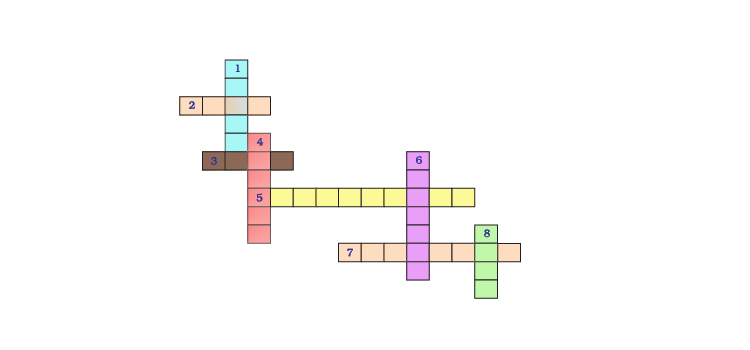Soln:46. (a) In this crossword puzzle (Fig 3.2), names of 11 elements are hidden. Symbols of these are given below. Complete the puzzle. 1. Cl 7. He 2. H 8. F 3. Ar 9. Kr 4. O 10. Rn 5. Xe 11. Ne 6. N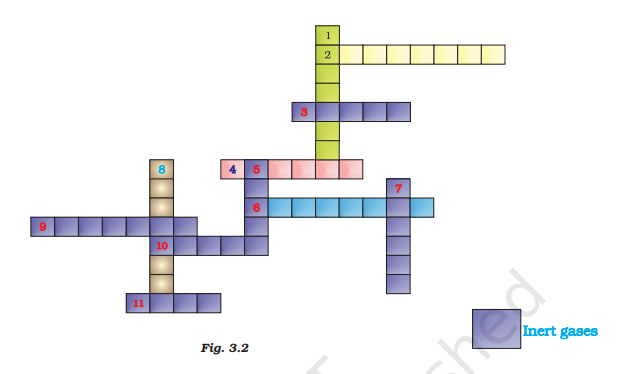Soln: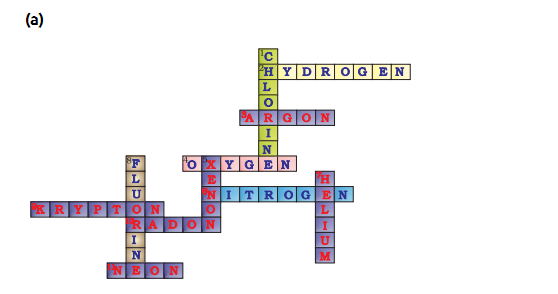b) Six : Helium (He); Neon ( Ne); Argon (Ar); Krypton (Kr); Xenon (Xe); Radon (Rn).

47. Write the formulae for the following and calculate the molecular mass for each one of them.

(a) Caustic potash

(b) Baking powder

(c) Limestone

(d) Caustic soda

(e) Ethanol

(f) Common salt

Soln:

The formulae for the following and calculate the molecular mass for each one of them.

 Ser. No. Compound Formula Molecular mass A Caustic Potash KOH 39+16+1=56u B Baking powder NaHCO3 23+1+12+3×16=84u C Limestone CaCO3 40+12+3×16=100u D Caustic soda NaOH 23+16+1=40u E Ethanol C2H5OH 2×2+5×1+16+1=46u F Common Salt NaCl 23+35.5=58.5

48. In photosynthesis, 6 molecules of carbon dioxide combine with an equal number of water molecules through a complex series of reactions to give a molecule of glucose having a molecular formula C6H12O6. How many grams of water would be required to produce 18 g of glucose? Compute the volume of water so consumed assuming the density of water to be 1 g cm-3.

Soln:

6CO2 + 6 H2 O Chlorophyll /Sunlight → C6 H12 O6 + 6O2

1 mole of glucose needs 6 moles of water  180 g of glucose needs (6×18) g of water  1 g of glucose will need 108/ 180 g of water.

18 g of glucose would need (108 /180) × 18 g of water = 10.8 g

Volume of water used = Mass  / Density

= 10.8 g/ 1g cm-3

= 10.8 cm3

### NCERT Exemplar for Class 9 Science Atoms and Molecules

Atoms and molecules are one of the most important chapters for CBSE Class 9. In this chapter, the students will learn some important concepts of chemistry like the law of conservation of mass, the law of constant proportions, atomic mass, what a molecule is and how to write a chemical formula. To practice higher-level questions from this chapter, students are advised to follow NCERT Exemplar Science Class 9 Chapter 3 Atoms and Molecules.

NCERT Exemplar for Class 9 Science Atoms and Molecules Important Concepts

1. Laws Of Chemical Combination (1a) Law of Conservation Of Mass (1b) Law of Constant Proportions
2. What Is An Atom? (2a) What Are the Modern Day Symbols of Atoms of Different Elements? (2b) Atomic Mass (2c) How Do Atoms Exist
3. What Is A Molecule? (3a) Molecules of Elements (3b) Molecules of Compounds (3c) What Is an Ion?
4. Writing Chemical Formulae (4a) Formulae of Simple Compounds
5. Molecular Mass And Mole Concept (5a) Molecular Mass Ex (5b) Formula Unit Mass (5c) Mole Concept

BYJU’S provides sample paper for Atoms and Molecules, previous year question papers, NCERT exemplars, worksheets exercises and assignments prepared by highly experienced teachers at BYJU’S. Students can easily access all the study material provided by us just by login into BYJU’S website or by downloading the BYJU’S Learning App.

## Frequently Asked Questions on NCERT Solutions for Class 10 Science Chapter 3

### Explain why the solubility of a substance changes with temperature as explained in Chapter 3 of NCERT Exemplar Solutions for Class 9 Science.

Solubility is the ability of a solute to get dissolved in 100g solvent. The solubility of a given solute to dissolve in a specific solvent depends on the temperature. With increase in temperature solubility of liquids and solids increase. In the same way solubility of gases decreases with increase in temperature.

### Mention the concepts explained in the Chapter 3 of NCERT Exemplar Solutions for Class 9 Science.

The concepts explained in the Chapter 3 of NCERT Exemplar Solutions for Class 9 Science are
1. Laws Of Chemical Combination
1a) Law of Conservation Of Mass
1b) Law of Constant Proportions
2. What Is An Atom?
(2a) What Are the Modern Day Symbols of Atoms of Different Elements?
(2b) Atomic Mass
(2c) How Do Atoms Exist
3. What Is A Molecule?
(3a) Molecules of Elements
(3b) Molecules of Compounds
(3c) What Is an Ion?
4. Writing Chemical Formulae
(4a) Formulae of Simple Compounds
5. Molecular Mass And Mole Concept
(5a) Molecular Mass Ex
(5b) Formula Unit Mass
(5c) Mole Concept

### Why should I use the NCERT Exemplar Solutions for Class 9 Science Chapter 3 while preparing for the exams?

The NCERT Exemplar Solutions for Class 9 Science Chapter 3 provides students with simple and step wise answers to the questions present in the textbook. The solutions are helpful to understand the method of answering the questions in the board exams. Students can save their time and fasten their revision which is very effective for exam preparation. The expert teachers at BYJU’S have designed the solutions based on the CBSE guidelines and syllabus.